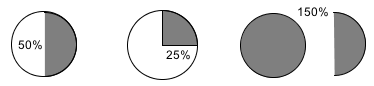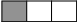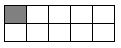### Home > AC > Chapter 1 > Lesson 1.1.2 > Problem1-13

1-13.

In Algebra, you will need to be able to work with numbers, words, and geometric representations. Use these representations to answer the following problems.

1. Draw and shade a figure that represents $100\%$. Label $100\%$ below it. Then describe the figure in words.

Diagrams will vary.

The figure can be described as a whole.2. Similarly, draw and shade figures that represent $50\%$, $25\%$, and $150\%$. Label each figure and describe it in words.$\frac{1}{3}$